# G(t): General description¶

## Purpose¶

Application to Analyze Stress Relaxation Data

## Data Files¶

• The first line of the file should contain the sample parameters separated by semi-colons (;). It may contain any number of parameters which will be read and saved as file-parameter in RepTate.

• Then the data columns should appear, separated by spaces or tabs.

### .gt extension¶

Text files with .gt extension should be organised as follows:

• .gt files should provide at least parameter values for

1. Molecular weight, $$M_w$$

2. Strain applied, $$\gamma$$. If not present, the experimental data file is supposed to contain the relaxation modulus, not the stress, and the value of $$\gamma$$ is assumed to be equal to 1.

• 2 columns separated by spaces or tabs containing respectively:

1. time, $$t$$,

2. shear stress, $$\sigma_{xy}$$,

A correct .gt file looks like:

Mw=224;gamma=1;
t sxy
0.0E+0      1.28146E+10
5.0E-6      1.13402E+10
1.0E-5      7.57171E+9
...         ...


## Views¶

### log[G(t)]¶

BaseApplicationGt.viewLogGt()[source]

Logarithm of the relaxation modulus $$G(t)$$ vs logarithm of time $$t$$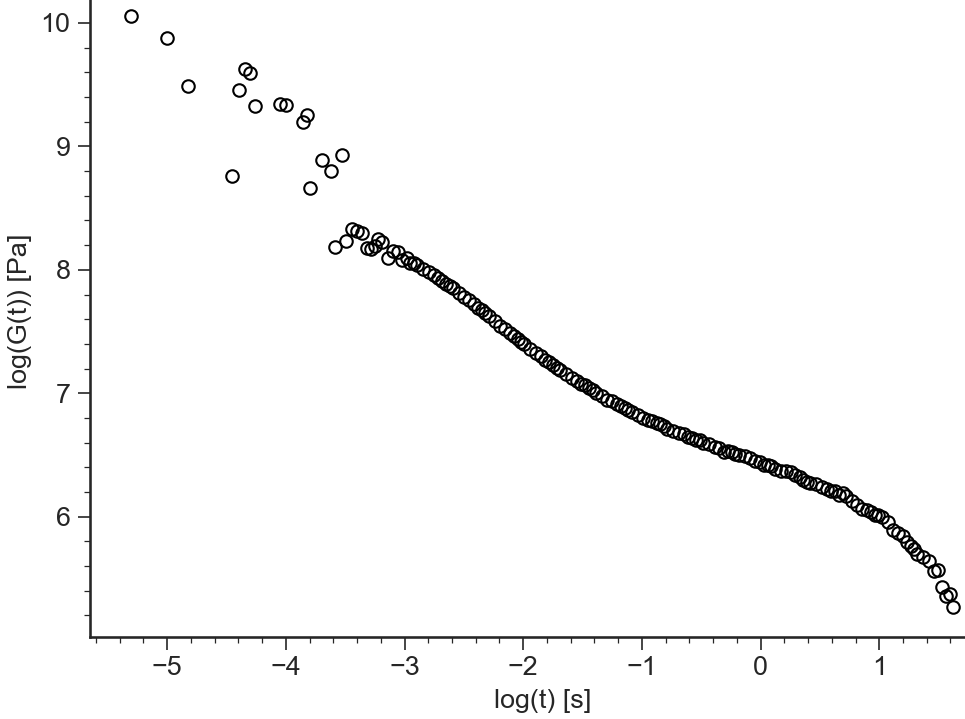### G(t)¶

BaseApplicationGt.viewGt()[source]

Relaxation modulus $$G(t)$$ vs time $$t$$ (both in logarithmic scale)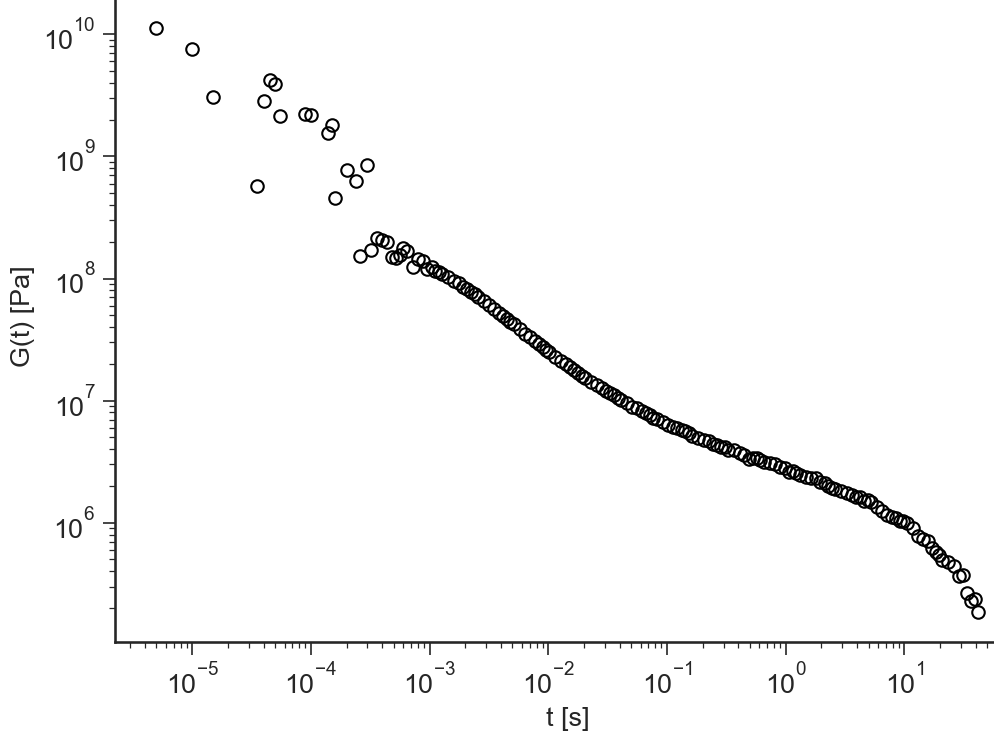### Schwarzl G’,G’’¶

BaseApplicationGt.viewSchwarzl_Gt()[source]

Schwarzl transformation: numerical calculation of the storage modulus $$G'(\omega)$$ and loss modulus $$G''(\omega)$$ from the relaxation modulus $$G(t)$$

The time range of the $$G(t)$$ that will be used for the Fourier transformation can be selected by changing the values of the text boxes $$\log(t_{min})$$ and $$\log(t_{min})$$. For more details, check .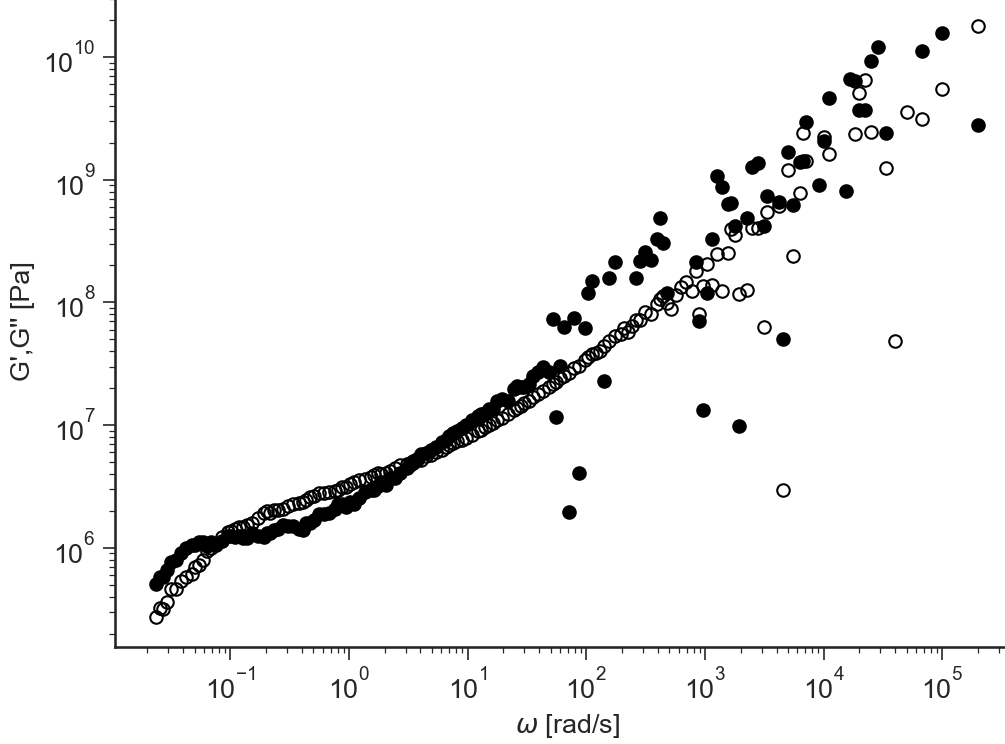### i-Rheo G’,G’’¶

BaseApplicationGt.viewiRheo()[source]

i-Rheo Fourier transformation of the relaxation modulus $$G(t)$$ to obtain the storage modulus $$G'(\omega)$$ and loss modulus $$G''(\omega)$$ (no oversamplig).

The time range of the $$G(t)$$ that will be used for the Fourier transformation can be selected by changing the values of the text boxes $$\log(t_{min})$$ and $$\log(t_{min})$$. For more details, check .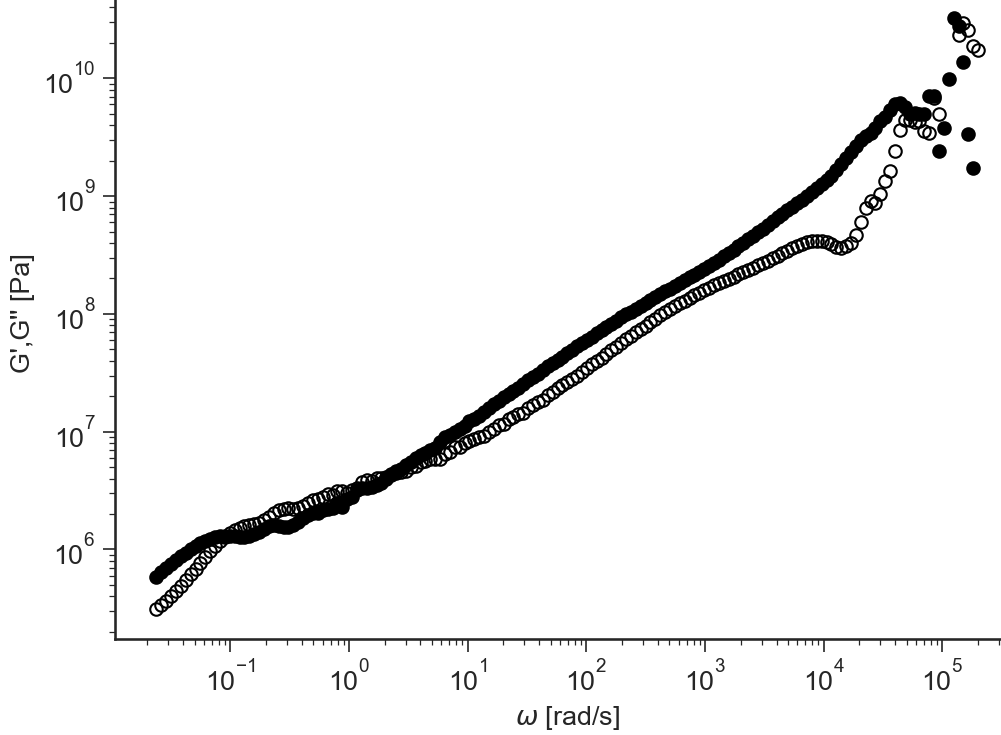### i-Rheo-Over G’,G’’¶

BaseApplicationGt.viewiRheoOver()[source]

i-Rheo Fourier transformation of the relaxation modulus $$G(t)$$ to obtain the storage modulus $$G'(\omega)$$ and loss modulus $$G''(\omega)$$ (with user selected oversamplig).

The time range of the $$G(t)$$ that will be used for the Fourier transformation can be selected by changing the values of the text boxes $$\log(t_{min})$$ and $$\log(t_{min})$$. For more details, check .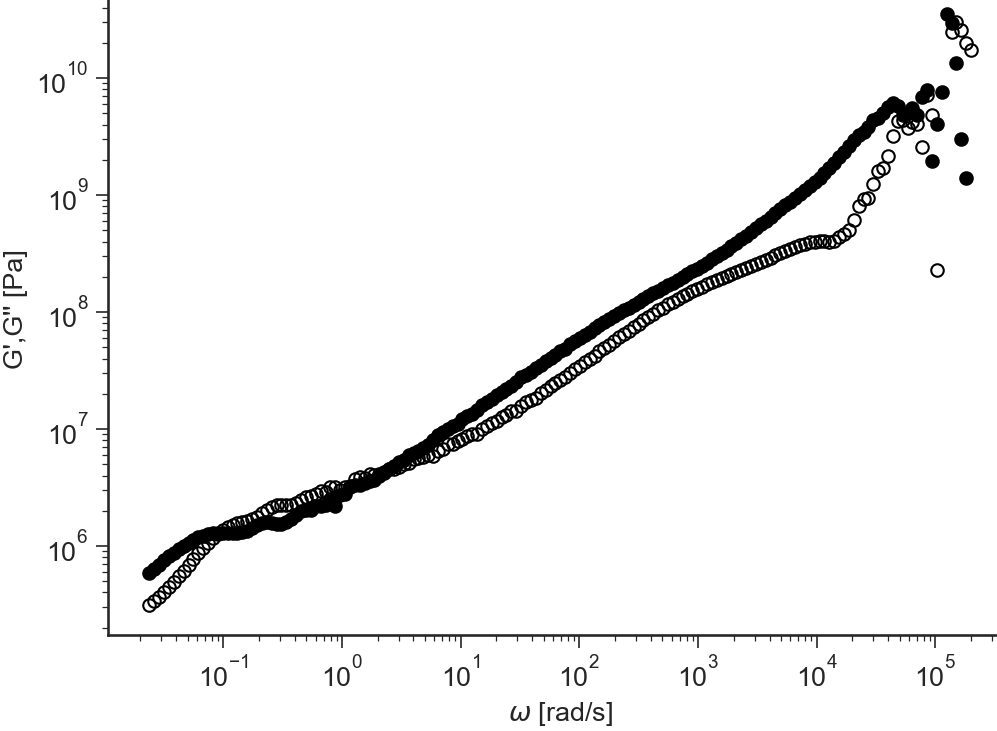References

1

F. R. Schwarzl. Numerical calculation of storage and loss modulus from stress relaxation data for linear viscoelastic materials. Rheologica Acta, 10(2):165–173, jun 1971. doi:10.1007/bf02040437.

2(1,2)

Manlio Tassieri, Marco Laurati, Dan J. Curtis, Dietmar W. Auhl, Salvatore Coppola, Andrea Scalfati, Karl Hawkins, Phylip Rhodri Williams, and Jonathan M. Cooper. I-rheo: measuring the materials\textquotesingle linear viscoelastic properties \textquotedblleft in a step\textquotedblright ! Journal of Rheology, 60(4):649–660, jul 2016. doi:10.1122/1.4953443.